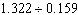Name:    Quiz 3.4-3.5

Multiple Choice
Identify the choice that best completes the statement or answers the question.

1.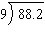a. 9.7 c. 98.1 b. 9.8 d. 98

2.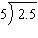a. 5 c. 0.5 b. 5.1 d. 0.4

3.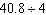a. 10.1 c. 102.1 b. 10.2 d. 102

4.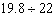a. 9.1 c. 9 b. 0.9 d. 0.8

5.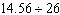a. 5.7 c. 0.46 b. 5.6 d. 0.56

6.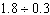a. 60 c. 5.9 b. 6.1 d. 6

7.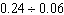a. 3.9 c. 40 b. 4 d. 4.1

8.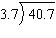a. 11.1 c. 10.9 b. 110 d. 11

9.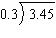a. 11.6 c. 11.4 b. 115 d. 11.5

10.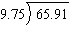a. 67.6 c. 6.66 b. 6.86 d. 6.76

11.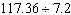a. 16.3 c. 163 b. 16.2 d. 16.4

12.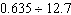a. 0.05 c. 0.06 b. 0.5 d. 0.04

Numeric Response

Evaluate the expression.

1.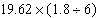Divide. Round to the nearest hundredth if necessary.

2.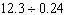1.

A 2-ounce bottle of suntan lotion costs \$3.06. A 6-ounce bottle costs \$9.48. Which is the better buy? Explain.

Without finding the quotient, complete the statement using <, >, or =. Explain your reasoning.

2.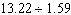?